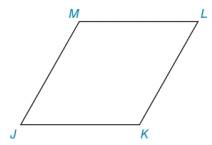Chapter 7.3, Problem 3EElementary Geometry For College St...

7th Edition
Alexander + 2 others
ISBN: 9781337614085

Solutions

Chapter
SectionElementary Geometry For College St...

7th Edition
Alexander + 2 others
ISBN: 9781337614085
Textbook Problem

Describe, if possible, how you would inscribe a circle in rhombus JKLM.To determine

To find:

The procedure to draw a circle inscribe a rhombus.

Explanation

Steps of construction to draw a circle inscribe a rhombus.

1) Construct the angle bisectors of LandK which intersect each other at O.

2) Draw a perpendicular from the centre O to side ML i.e., OP.

Still sussing out bartleby?

Check out a sample textbook solution.

See a sample solution

The Solution to Your Study Problems

Bartleby provides explanations to thousands of textbook problems written by our experts, many with advanced degrees!

Get Started

Calculate y'. 8. xey = y sin x

Single Variable Calculus: Early Transcendentals, Volume I

Evaluate the integral. 42. sin2sin6d

Single Variable Calculus: Early Transcendentals

7. List four perils covered by property insurance. (19-4)

Contemporary Mathematics for Business & Consumers

The slope of the line tangent to the circle x2 + y2 = 100 at the point (6, 8) is: a) 34 b) 34 c) 43 d) 43

Study Guide for Stewart's Single Variable Calculus: Early Transcendentals, 8th

For f(x, y) = ln(x + y), f(e2, e3) = 2 ln (1 + e) 2 + ln(1 + e) 5

Study Guide for Stewart's Multivariable Calculus, 8th